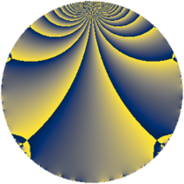# Properties

 Label 245.2.aLevel $245$ Weight $2$ Character orbit 245.a Rep. character $\chi_{245}(1,\cdot)$ Character field $\Q$ Dimension $13$ Newform subspaces $8$ Sturm bound $56$ Trace bound $3$

# Related objects

## Defining parameters

 Level: $$N$$ $$=$$ $$245 = 5 \cdot 7^{2}$$ Weight: $$k$$ $$=$$ $$2$$ Character orbit: $$[\chi]$$ $$=$$ 245.a (trivial) Character field: $$\Q$$ Newform subspaces: $$8$$ Sturm bound: $$56$$ Trace bound: $$3$$ Distinguishing $$T_p$$: $$2$$, $$3$$

## Dimensions

The following table gives the dimensions of various subspaces of $$M_{2}(\Gamma_0(245))$$.

Total New Old
Modular forms 36 13 23
Cusp forms 21 13 8
Eisenstein series 15 0 15

The following table gives the dimensions of the cuspidal new subspaces with specified eigenvalues for the Atkin-Lehner operators and the Fricke involution.

$$5$$$$7$$FrickeDim.
$$+$$$$+$$$$+$$$$2$$
$$+$$$$-$$$$-$$$$5$$
$$-$$$$+$$$$-$$$$4$$
$$-$$$$-$$$$+$$$$2$$
Plus space$$+$$$$4$$
Minus space$$-$$$$9$$

## Trace form

 $$13 q - q^{2} + 11 q^{4} - q^{5} + 8 q^{6} + 3 q^{8} + 13 q^{9} + O(q^{10})$$ $$13 q - q^{2} + 11 q^{4} - q^{5} + 8 q^{6} + 3 q^{8} + 13 q^{9} + q^{10} - 4 q^{11} - 4 q^{12} - 10 q^{13} - 5 q^{16} + 2 q^{17} - 17 q^{18} + 4 q^{19} - 7 q^{20} - 20 q^{22} + 4 q^{23} + 4 q^{24} + 13 q^{25} - 6 q^{26} + 12 q^{27} - 14 q^{29} + 8 q^{30} + 4 q^{31} - 5 q^{32} + 12 q^{33} + 6 q^{34} - 5 q^{36} + 6 q^{37} - 20 q^{38} + 24 q^{39} + 9 q^{40} + 10 q^{41} + 16 q^{43} - 20 q^{44} - 5 q^{45} - 4 q^{46} - 4 q^{47} - 28 q^{48} - q^{50} - 40 q^{51} + 6 q^{52} - 26 q^{53} - 12 q^{54} - 4 q^{55} + 32 q^{57} + 6 q^{58} + 8 q^{59} - 16 q^{60} - 14 q^{61} - 13 q^{64} - 2 q^{65} + 4 q^{66} + 10 q^{68} - 12 q^{69} - 40 q^{71} - 53 q^{72} + 6 q^{73} - 30 q^{74} + 36 q^{76} - 32 q^{78} + 8 q^{79} + q^{80} - 3 q^{81} + 18 q^{82} - 20 q^{83} + 2 q^{85} + 48 q^{86} - 28 q^{87} + 44 q^{88} + 6 q^{89} - 7 q^{90} + 20 q^{92} + 8 q^{93} - 28 q^{94} + 4 q^{95} + 4 q^{96} + 10 q^{97} + 28 q^{99} + O(q^{100})$$

## Decomposition of $$S_{2}^{\mathrm{new}}(\Gamma_0(245))$$ into newform subspaces

Label Dim $A$ Field CM Traces A-L signs $q$-expansion
$a_{2}$ $a_{3}$ $a_{5}$ $a_{7}$ 5 7
245.2.a.a $1$ $1.956$ $$\Q$$ None $$-2$$ $$-3$$ $$1$$ $$0$$ $-$ $-$ $$q-2q^{2}-3q^{3}+2q^{4}+q^{5}+6q^{6}+\cdots$$
245.2.a.b $1$ $1.956$ $$\Q$$ None $$-2$$ $$3$$ $$-1$$ $$0$$ $+$ $-$ $$q-2q^{2}+3q^{3}+2q^{4}-q^{5}-6q^{6}+\cdots$$
245.2.a.c $1$ $1.956$ $$\Q$$ None $$0$$ $$-1$$ $$1$$ $$0$$ $-$ $-$ $$q-q^{3}-2q^{4}+q^{5}-2q^{9}-3q^{11}+\cdots$$
245.2.a.d $2$ $1.956$ $$\Q(\sqrt{17})$$ None $$-1$$ $$1$$ $$-2$$ $$0$$ $+$ $-$ $$q-\beta q^{2}+(1-\beta )q^{3}+(2+\beta )q^{4}-q^{5}+\cdots$$
245.2.a.e $2$ $1.956$ $$\Q(\sqrt{2})$$ None $$0$$ $$-2$$ $$-2$$ $$0$$ $+$ $+$ $$q+\beta q^{2}+(-1-\beta )q^{3}-q^{5}+(-2-\beta )q^{6}+\cdots$$
245.2.a.f $2$ $1.956$ $$\Q(\sqrt{2})$$ None $$0$$ $$2$$ $$2$$ $$0$$ $-$ $+$ $$q+\beta q^{2}+(1+\beta )q^{3}+q^{5}+(2+\beta )q^{6}+\cdots$$
245.2.a.g $2$ $1.956$ $$\Q(\sqrt{2})$$ None $$2$$ $$-2$$ $$-2$$ $$0$$ $+$ $-$ $$q+(1+\beta )q^{2}+(-1+\beta )q^{3}+(1+2\beta )q^{4}+\cdots$$
245.2.a.h $2$ $1.956$ $$\Q(\sqrt{2})$$ None $$2$$ $$2$$ $$2$$ $$0$$ $-$ $+$ $$q+(1+\beta )q^{2}+(1-\beta )q^{3}+(1+2\beta )q^{4}+\cdots$$

## Decomposition of $$S_{2}^{\mathrm{old}}(\Gamma_0(245))$$ into lower level spaces

$$S_{2}^{\mathrm{old}}(\Gamma_0(245)) \cong$$ $$S_{2}^{\mathrm{new}}(\Gamma_0(35))$$$$^{\oplus 2}$$$$\oplus$$$$S_{2}^{\mathrm{new}}(\Gamma_0(49))$$$$^{\oplus 2}$$